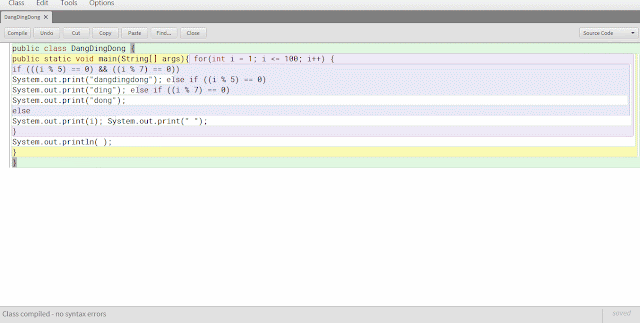FOR statement JAVA

The loop process (Loop: FOR statement)

An iterative process using the statement for the java language you can see the program coding examples below:
 `01` `public ``class` `GalToLiter{`
 `02` `public static void main(String args[]) {`
 `03` `double liter;`
 `04` `int` `galon;`
 `05` `int` `counter; counter ``=` `0``;`
 `06` `for``(galon ``=` `1``; galon <``=` `100``; galon``+``+``){ liter ``=` `galon ``*` `3.7854``;`
 `07` `System.out.println(galon ``+` `" galon adalah "` `+` `liter ``+` `" liter."``); counter``+``+``;`
 `08` `if``(counter ``=``=` `10``) {`
 `09` `System.out.println(); counter ``=` `0``;`
 `10` `}`
 `11` `}`
 `12` `}`
 `13` `}`coding results, will produce results loping of charging each additional gallon, every gallon added the liter will increase up to 100 gallons.

Examples of the other looping coding is dangdingdong following games:
 `01` `public ``class` `DangDingDong {`
 `02` `public static void main(String[] args){ ``for``(``int` `i ``=` `1``; i <``=` `100``; i``+``+``) {`
 `03` `if` `(((i ``%` `5``) ``=``=` `0``) && ((i ``%` `7``) ``=``=` `0``))`
 `04` `System.out.``print``(``"dangdingdong"``); ``else` `if` `((i ``%` `5``) ``=``=` `0``)`
 `05` `System.out.``print``(``"ding"``); ``else` `if` `((i ``%` `7``) ``=``=` `0``)`
 `06` `System.out.``print``(``"dong"``);`
 `07` `else`
 `08` `System.out.``print``(i); System.out.``print``(``" "``);`
 `09` `}`
 `10` `System.out.println( );`
 `11` `}`
 `12` `}`coding results from this program produces looping to the provisions making the numbers 1 to 100, wherein each multiple of five will bring up the word ding, each multiple of 7 displays the word dong, and if the number of multiples of 5 and 7 simultaneously unite as number 35 will display the dangdingdong.

Examples of coding looping dangdingdong game with Switch:
 `01` `public ``class` `dangdingdongSwitch {`
 `02` `public static void main(String[] args) { ``for``(``int` `i ``=` `1``; i <``=` `100``; i``+``+``) {`
 `03` `switch(i ``%` `35``) { case ``0``:`
 `04` `System.out.``print``(``"DangDingDong "``); ``break``;`
 `05` `case ``5``:`
 `06` `case ``10``:`
 `07` `case ``15``:`
 `08` `case ``20``:`
 `09` `case ``25``:`
 `10` `case ``30``:`
 `11` `System.out.``print``(``"Ding "``); ``break``;`
 `12` `case ``7``:`
 `13` `case ``14``:`
 `14` `case ``21``:`
 `15` `case ``28``:`
 `16` `System.out.``print``(``"Dong "``); ``break``;`
 `17` `default:`
 `18` `System.out.``print``(i ``+` `" "``); ``break``;`
 `19` `}`
 `20` `}`
 `21` `System.out.println( );`
 `22` `}`
 `23` `}`the results of coding, the same as the previous examples in this program differ only in the increments are using a switch case

Berlangganan update artikel terbaru via email: